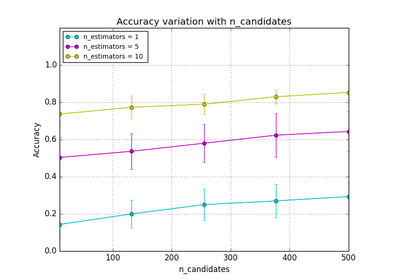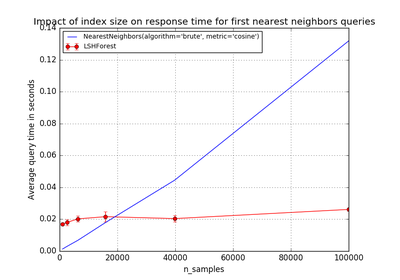# `sklearn.neighbors`.NearestNeighbors¶

class `sklearn.neighbors.``NearestNeighbors`(n_neighbors=5, radius=1.0, algorithm='auto', leaf_size=30, metric='minkowski', p=2, metric_params=None, n_jobs=1, **kwargs)[source]

Unsupervised learner for implementing neighbor searches.

Read more in the User Guide.

Parameters: n_neighbors : int, optional (default = 5) Number of neighbors to use by default for `k_neighbors` queries. radius : float, optional (default = 1.0) Range of parameter space to use by default for `radius_neighbors` queries. algorithm : {‘auto’, ‘ball_tree’, ‘kd_tree’, ‘brute’}, optional Algorithm used to compute the nearest neighbors: ‘ball_tree’ will use `BallTree` ‘kd_tree’ will use `KDtree` ‘brute’ will use a brute-force search. ‘auto’ will attempt to decide the most appropriate algorithm based on the values passed to `fit` method. Note: fitting on sparse input will override the setting of this parameter, using brute force. leaf_size : int, optional (default = 30) Leaf size passed to BallTree or KDTree. This can affect the speed of the construction and query, as well as the memory required to store the tree. The optimal value depends on the nature of the problem. p: integer, optional (default = 2) : Parameter for the Minkowski metric from sklearn.metrics.pairwise.pairwise_distances. When p = 1, this is equivalent to using manhattan_distance (l1), and euclidean_distance (l2) for p = 2. For arbitrary p, minkowski_distance (l_p) is used. metric : string or callable, default ‘minkowski’ metric to use for distance computation. Any metric from scikit-learn or scipy.spatial.distance can be used. If metric is a callable function, it is called on each pair of instances (rows) and the resulting value recorded. The callable should take two arrays as input and return one value indicating the distance between them. This works for Scipy’s metrics, but is less efficient than passing the metric name as a string. Distance matrices are not supported. Valid values for metric are: from scikit-learn: [‘cityblock’, ‘cosine’, ‘euclidean’, ‘l1’, ‘l2’, ‘manhattan’] from scipy.spatial.distance: [‘braycurtis’, ‘canberra’, ‘chebyshev’, ‘correlation’, ‘dice’, ‘hamming’, ‘jaccard’, ‘kulsinski’, ‘mahalanobis’, ‘matching’, ‘minkowski’, ‘rogerstanimoto’, ‘russellrao’, ‘seuclidean’, ‘sokalmichener’, ‘sokalsneath’, ‘sqeuclidean’, ‘yule’] See the documentation for scipy.spatial.distance for details on these metrics. metric_params : dict, optional (default = None) Additional keyword arguments for the metric function. n_jobs : int, optional (default = 1) The number of parallel jobs to run for neighbors search. If `-1`, then the number of jobs is set to the number of CPU cores. Affects only `k_neighbors` and `kneighbors_graph` methods.

Notes

See Nearest Neighbors in the online documentation for a discussion of the choice of `algorithm` and `leaf_size`.

https://en.wikipedia.org/wiki/K-nearest_neighbor_algorithm

Examples

```>>> import numpy as np
>>> from sklearn.neighbors import NearestNeighbors
>>> samples = [[0, 0, 2], [1, 0, 0], [0, 0, 1]]
```
```>>> neigh = NearestNeighbors(2, 0.4)
>>> neigh.fit(samples)
NearestNeighbors(...)
```
```>>> neigh.kneighbors([[0, 0, 1.3]], 2, return_distance=False)
...
array([[2, 0]]...)
```
```>>> nbrs = neigh.radius_neighbors([[0, 0, 1.3]], 0.4, return_distance=False)
>>> np.asarray(nbrs)
array(2)
```

Methods

 `fit`(X[, y]) Fit the model using X as training data `get_params`([deep]) Get parameters for this estimator. `kneighbors`([X, n_neighbors, return_distance]) Finds the K-neighbors of a point. `kneighbors_graph`([X, n_neighbors, mode]) Computes the (weighted) graph of k-Neighbors for points in X `radius_neighbors`([X, radius, return_distance]) Finds the neighbors within a given radius of a point or points. `radius_neighbors_graph`([X, radius, mode]) Computes the (weighted) graph of Neighbors for points in X `set_params`(\*\*params) Set the parameters of this estimator.
`__init__`(n_neighbors=5, radius=1.0, algorithm='auto', leaf_size=30, metric='minkowski', p=2, metric_params=None, n_jobs=1, **kwargs)[source]
`fit`(X, y=None)[source]

Fit the model using X as training data

Parameters: X : {array-like, sparse matrix, BallTree, KDTree} Training data. If array or matrix, shape [n_samples, n_features], or [n_samples, n_samples] if metric=’precomputed’.
`get_params`(deep=True)[source]

Get parameters for this estimator.

Parameters: deep : boolean, optional If True, will return the parameters for this estimator and contained subobjects that are estimators. params : mapping of string to any Parameter names mapped to their values.
`kneighbors`(X=None, n_neighbors=None, return_distance=True)[source]

Finds the K-neighbors of a point.

Returns indices of and distances to the neighbors of each point.

Parameters: X : array-like, shape (n_query, n_features), or (n_query, n_indexed) if metric == ‘precomputed’ The query point or points. If not provided, neighbors of each indexed point are returned. In this case, the query point is not considered its own neighbor. n_neighbors : int Number of neighbors to get (default is the value passed to the constructor). return_distance : boolean, optional. Defaults to True. If False, distances will not be returned dist : array Array representing the lengths to points, only present if return_distance=True ind : array Indices of the nearest points in the population matrix.

Examples

In the following example, we construct a NeighborsClassifier class from an array representing our data set and ask who’s the closest point to [1,1,1]

```>>> samples = [[0., 0., 0.], [0., .5, 0.], [1., 1., .5]]
>>> from sklearn.neighbors import NearestNeighbors
>>> neigh = NearestNeighbors(n_neighbors=1)
>>> neigh.fit(samples)
NearestNeighbors(algorithm='auto', leaf_size=30, ...)
>>> print(neigh.kneighbors([[1., 1., 1.]]))
(array([[ 0.5]]), array([]...))
```

As you can see, it returns [[0.5]], and [], which means that the element is at distance 0.5 and is the third element of samples (indexes start at 0). You can also query for multiple points:

```>>> X = [[0., 1., 0.], [1., 0., 1.]]
>>> neigh.kneighbors(X, return_distance=False)
array([,
]...)
```
`kneighbors_graph`(X=None, n_neighbors=None, mode='connectivity')[source]

Computes the (weighted) graph of k-Neighbors for points in X

Parameters: X : array-like, shape (n_query, n_features), or (n_query, n_indexed) if metric == ‘precomputed’ The query point or points. If not provided, neighbors of each indexed point are returned. In this case, the query point is not considered its own neighbor. n_neighbors : int Number of neighbors for each sample. (default is value passed to the constructor). mode : {‘connectivity’, ‘distance’}, optional Type of returned matrix: ‘connectivity’ will return the connectivity matrix with ones and zeros, in ‘distance’ the edges are Euclidean distance between points. A : sparse matrix in CSR format, shape = [n_samples, n_samples_fit] n_samples_fit is the number of samples in the fitted data A[i, j] is assigned the weight of edge that connects i to j.

Examples

```>>> X = [, , ]
>>> from sklearn.neighbors import NearestNeighbors
>>> neigh = NearestNeighbors(n_neighbors=2)
>>> neigh.fit(X)
NearestNeighbors(algorithm='auto', leaf_size=30, ...)
>>> A = neigh.kneighbors_graph(X)
>>> A.toarray()
array([[ 1.,  0.,  1.],
[ 0.,  1.,  1.],
[ 1.,  0.,  1.]])
```
`radius_neighbors`(X=None, radius=None, return_distance=True)[source]

Finds the neighbors within a given radius of a point or points.

Return the indices and distances of each point from the dataset lying in a ball with size `radius` around the points of the query array. Points lying on the boundary are included in the results.

The result points are not necessarily sorted by distance to their query point.

Parameters: X : array-like, (n_samples, n_features), optional The query point or points. If not provided, neighbors of each indexed point are returned. In this case, the query point is not considered its own neighbor. radius : float Limiting distance of neighbors to return. (default is the value passed to the constructor). return_distance : boolean, optional. Defaults to True. If False, distances will not be returned dist : array, shape (n_samples,) of arrays Array representing the distances to each point, only present if return_distance=True. The distance values are computed according to the `metric` constructor parameter. ind : array, shape (n_samples,) of arrays An array of arrays of indices of the approximate nearest points from the population matrix that lie within a ball of size `radius` around the query points.

Notes

Because the number of neighbors of each point is not necessarily equal, the results for multiple query points cannot be fit in a standard data array. For efficiency, radius_neighbors returns arrays of objects, where each object is a 1D array of indices or distances.

Examples

In the following example, we construct a NeighborsClassifier class from an array representing our data set and ask who’s the closest point to [1, 1, 1]:

```>>> import numpy as np
>>> samples = [[0., 0., 0.], [0., .5, 0.], [1., 1., .5]]
>>> from sklearn.neighbors import NearestNeighbors
>>> neigh.fit(samples)
NearestNeighbors(algorithm='auto', leaf_size=30, ...)
>>> rng = neigh.radius_neighbors([[1., 1., 1.]])
>>> print(np.asarray(rng))
[ 1.5  0.5]
>>> print(np.asarray(rng))
[1 2]
```

The first array returned contains the distances to all points which are closer than 1.6, while the second array returned contains their indices. In general, multiple points can be queried at the same time.

`radius_neighbors_graph`(X=None, radius=None, mode='connectivity')[source]

Computes the (weighted) graph of Neighbors for points in X

Neighborhoods are restricted the points at a distance lower than radius.

Parameters: X : array-like, shape = [n_samples, n_features], optional The query point or points. If not provided, neighbors of each indexed point are returned. In this case, the query point is not considered its own neighbor. radius : float Radius of neighborhoods. (default is the value passed to the constructor). mode : {‘connectivity’, ‘distance’}, optional Type of returned matrix: ‘connectivity’ will return the connectivity matrix with ones and zeros, in ‘distance’ the edges are Euclidean distance between points. A : sparse matrix in CSR format, shape = [n_samples, n_samples] A[i, j] is assigned the weight of edge that connects i to j.

Examples

```>>> X = [, , ]
>>> from sklearn.neighbors import NearestNeighbors
>>> neigh.fit(X)
NearestNeighbors(algorithm='auto', leaf_size=30, ...)
>>> A.toarray()
array([[ 1.,  0.,  1.],
[ 0.,  1.,  0.],
[ 1.,  0.,  1.]])
```
`set_params`(**params)[source]

Set the parameters of this estimator.

The method works on simple estimators as well as on nested objects (such as pipelines). The latter have parameters of the form `<component>__<parameter>` so that it’s possible to update each component of a nested object.

Returns: self :

## Examples using `sklearn.neighbors.NearestNeighbors`¶Hyper-parameters of Approximate Nearest NeighborsScalability of Approximate Nearest Neighbors